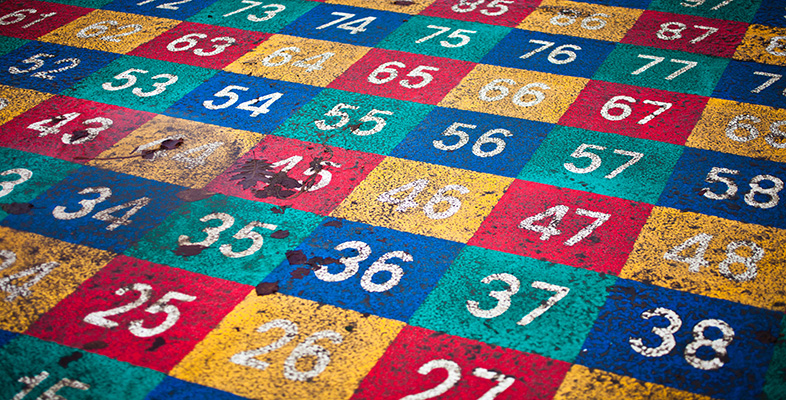Complex numbers

This free course is available to start right now. Review the full course description and key learning outcomes and create an account and enrol if you want a free statement of participation.

Free course

# 4.4 Self-assessment questions and problems

## SAQ 26

Find the distance between the numbers 2 − i and 1 + 3i.

### Answer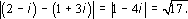## SAQ 26

In each of the following cases, classify the statement as True or False.

(i) The set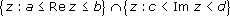, where a, b, c, dR, is a rectangle.

(ii) The set in (i) is a closed rectangle

(iii) The set in (i) is an open rectangle.

(iv) The set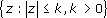is a disc

(v) The set in (iv) is a closed disc.

(vi) The sets Sn, n = 1, 2, 3 …, where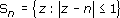form a nested sequence.

(vii) The sets Sn, n = 1, 2, 3…, where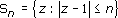form a nested sequence.

(viii) The sets Sn, n = 1, 2, 3…, where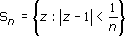form a nested sequence.

(ix) The sets Sn, n = 1, 2, 3…, where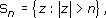form a nested sequence.

### Answer

(i) True.

(ii) False.

(iii) False.

(iv) True.

(v) True.

(vi) False (Figure 27).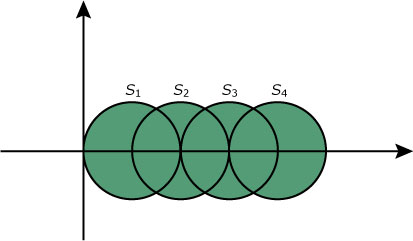Figure 27

(vii) False (Figure 28).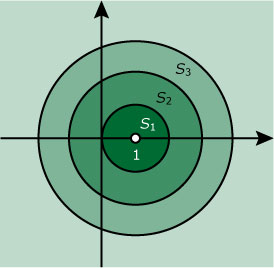Figure 28

(viii) True (Figure 29).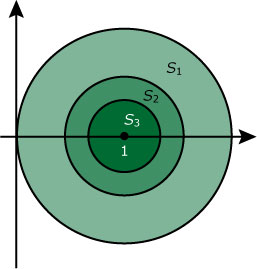Figure 29

(ix) True (Figure 30).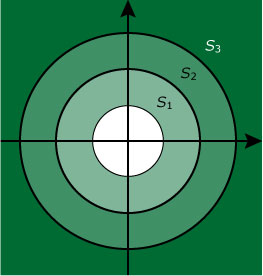Figure 30

## SAQ 27

Sketch the curves corresponding to the following equations.

(i) |z + 1 | = 1.

(ii) |z + 3−i| = |z−2 |.

### Answer

(i)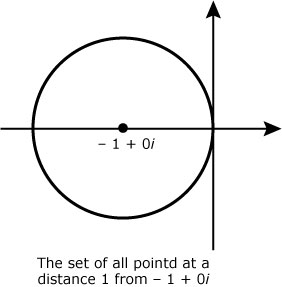Figure 31

(ii)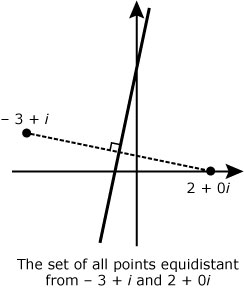Figure 32

## SAQ 28

Find the Cartesian equation (that is an equation connecting x and y directly) of each of the curves in SAQ 27.

### Answer

(i) (x + 1)2 + y2 = 1.

(ii) 5xy + 3 = 0.

## SAQ 29

If you have ever tried to cut a flower bed by tying the ends of a piece of string to two canes so that the string is slack and then fiddling about with another cane keeping the string taut, as in Figure 33, then you will know that the equation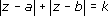is the equation of an ellipse. Find the Cartesian form of the equation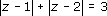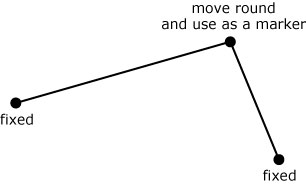Figure 33

### Answer

You have to tread with caution to avoid as much arithmetic as possible. One way is to write

| z−1 | = 3−| z−2 |

and so

| z−1 |2 = 9−6 | z−2 | + | z + 2 |2

giving

36 | z−2 |2 =  ( 9  + | z−2 |2−| z−1 |2)2.

The right-hand side simplifies considerably and (after a few days’ work) you should arrive at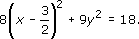(It is possible to go straight to x and y in the first equation, but in initial manipulations it is often simpler to work in terms of z.)

## SAQ 30

Sketch each of the following sets of points, where a, b,, c, dC: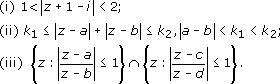### Answer

(i)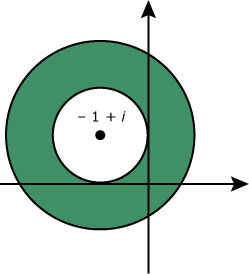Figure 34

(ii)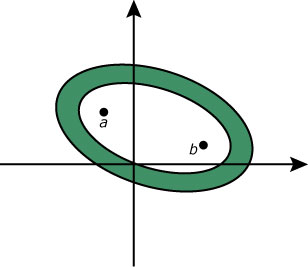Figure 35

(iii)Figure 36

(Note that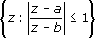is the set of all points whose distance from a is less than or equal to their distance from b.)

## SAQ 31

(i) We sometimes want an algebraic description of a line segment in the complex plane, rather than a complete line. One way of doing this is to use a real parameter. Describe the setwhere t is real and z1 and z2 are fixed complex numbers. Identify the points corresponding to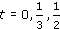and 1 respectively.

(ii) Describe the set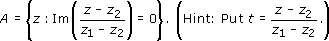### Answer

(i) The set is the set of points on the line segment joining z1 and z2, which is denoted by [z1, z2] by analogy with an interval on the real line. The points corresponding to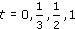correspond to the points: z2, the point of trisection of [z1, z2] nearer to z2, the midpoint, and z1, respectively.

(ii) Since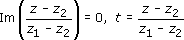is real and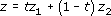. Hence A is the line which includes the line segment of part (i).

## SAQ 32

(i) Prove the following inequalities.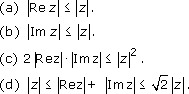(ii) Show that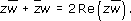(iii) Use the formula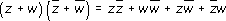together with part (ii) and a variation of (a) of part (i) to prove the triangle inequality: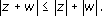(iv) By writing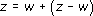, prove that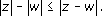Prove, similarly, that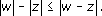and hence that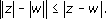Interpret this result geometrically.

### Answer

(i) (a)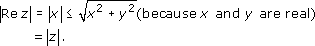(b)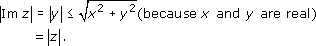(c) Since, for any real numbers x and y, we have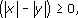we can deduce that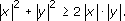and so the result follows.

(d) We have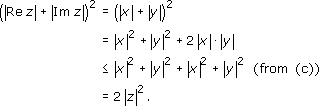Also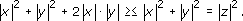So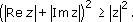The result follows because moduli are nonnegative.

(ii)For any complex number α,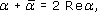( see Problem 3(i) of Section 1.4)

Put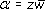and note that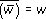; then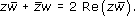(iii)We have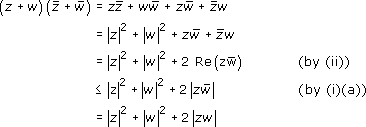Also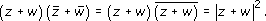Thus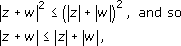since both sides are nonnegative.

(iv)From (iii), we have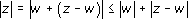. Thus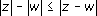. Interchanging z and w, gives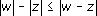and combining the two results givesThe result corresponds to the fact that the difference between the lengths of any two sides of a triangle does not exceed the length of the third side.

## SAQ 33

Show that the function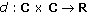where d(z,w) = |zw| is a distance function: that is to say d satisfies: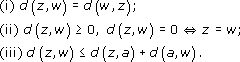### Answer

(i)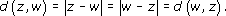(ii) If z = x + iy and w = u + iv then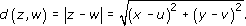Thus d(z, w) = 0 if and only if x= u and y = v; that is if z = w, d(z, w) > 0 otherwise, because |zw| is nonnegative.

(iii) We have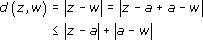using the result of SAQ 32 (iii), with z replaced by za and w replaced by aw. Thus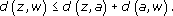(Note that the set C and the function d with domain C × C constitutes a metric space.)

## SAQ 34

Prove that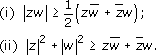### Answer

(i)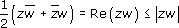by part (a) of SAQ 32 (i).

(ii) Since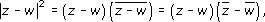we know that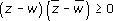and so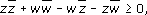from which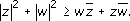## SAQ 35

Find an upper bound for each of ihe following sets.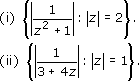### Answer

(i) We know that |z| = 2 and we want to replace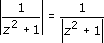by something larger. This means replacing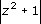by something smaller. We can write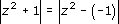and use the result of SAQ 32 (iv) to give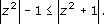So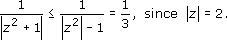(ii) A similar argument to that in (i) gives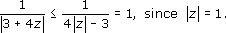The next problem deals with the Bolzano–Weierstrass Theorem. Bernard Bolzano (1781–1848) was professor of the Philosophy of Religion, in Prague. He wrote a treatise entitled Paradoxes of the Infinite, but this did not appear until after his death. Karl Weierstrass (1815–1897) was, together with Cauchy and Riemann, one of the founders of complex analysis. He led an uneventful life, much of it spent in Berlin where he was the professor of Mathematics. He is remembered both for his work on elliptic functions, and also for his attempts to put analysis on a firm logical foundation. You will come across his name in connection with the Bolzano–Weierstrass Theorem, the Casorati–Weierstrass Theorem, and the Weierstrass M-test for uniform convergence.

To pose this problem we require the following definition.

Definition

Let S be any set, and z0 any point in C. Then z0 is called a cluster point of S if for all> 0 there is zS such that. (Note that z0 need not belong to S.)

## SAQ 36

Prove the Bolzano–Weierstrass Theorem: if S is a bounded set and contains infinitely many complex numbers then S has at least one cluster point.

### Answer

Since S is bounded there is a closed disc with centre the origin and radius K, say, which contains S. Therefore there is a closed square, S0, with vertices at ±K ±iK which encloses S. The imaginary axis divides this square into two closed rectangles. At least one of these must contain infinitely many points. Choose this rectangle. If both rectangles contain infinitely many points, choose the right-hand one. The real axis divides this rectangle into two closed squares. At least one of these contains infinitely many points of S. Choose this one, or the upper one if they both do. Call this square S1. If we continue in this way, dividing each square by a vertical line and then by a horizontal line, we get a nested sequence of closed squares {Sn} where Sn has side of length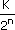.

Also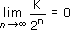. By the Nested Rectangles Theorem, there is one and only one point z0 in every square. This is a cluster point, because given> 0, the disc |zz0| <contains the squares Sn where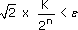, that is,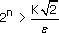(Figure 37). (Notice that z0 could be at the corner of Sn: this position giving the maximum size of disc required.) Thus the disc contains an infinite number of points of the set.

Notice that there may be more than one cluster point. The cluster point will be unique if at each stage of the process only one of the two rectangles contains an infinite number of points of S.Figure 37
M332_1

### Take your learning further

Making the decision to study can be a big step, which is why you'll want a trusted University. The Open University has 50 years’ experience delivering flexible learning and 170,000 students are studying with us right now. Take a look at all Open University courses.

If you are new to university level study, find out more about the types of qualifications we offer, including our entry level Access courses and Certificates.

Not ready for University study then browse over 900 free courses on OpenLearn and sign up to our newsletter to hear about new free courses as they are released.

Every year, thousands of students decide to study with The Open University. With over 120 qualifications, we’ve got the right course for you.

Request an Open University prospectus